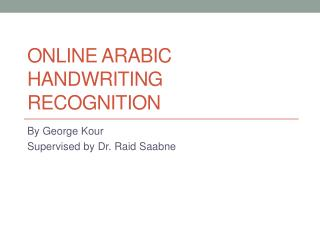Download PresentationOnline Arabic Handwriting Recognition

# Online Arabic Handwriting Recognition

Download Presentation## Online Arabic Handwriting Recognition

- - - - - - - - - - - - - - - - - - - - - - - - - - - E N D - - - - - - - - - - - - - - - - - - - - - - - - - - -
##### Presentation Transcript

1. Online Arabic Handwriting Recognition By George Kour Supervised by Dr. Raid Saabne

2. Machine Learning (Optional) • Main model (PAC)

3. Pattern Recognition(Optional) • Supervised learning vs. unsupervised learning • Classification techniques • Binary classification vs. multiclass classification • Naïve Bayes • Neural Network • Tree • Clustering • Supervised techniques • SVM • K- means

4. Background • Feature • Metrics • Dimensionality Reduction • Classification

5. The Arabic Letters • Arabic is the Mother tongue of more than 350 Million people. • Other languages that use the Arabic letters is parsian ... • How many manuscripts arte written in Arabic • Arabic is a cursive language • It is composed by word parts. • Show samples of Arabic script.

6. denotes +1 denotes -1 Support Vector Machines • Given Training sample data of the form: • Find the maximum margin hyperplabe that divides samples of the two classes. • The hyperplane formula: • If the samples are linearly separable, there may be infinite hyperplanes separating the samples of the two classes. Which is the best? x2 x1

7. denotes +1 denotes -1 x+ x+ x- Support Vectors Support Vector Machines x2 Margin • Minimize • We want to prevent data points falling into the margin, we add the following constraint: • Using the Langrange multipliers we obatin the quaqdratic optimization problem: wT x + b = 1 wT x + b = 0 wT x + b = -1 n x1

8. x 0 • But what are we going to do if the dataset is just too hard? x 0 x2 x 0 Non Linear SVM • Datasets that are linearly separable with noise work out great: • How about… mapping data to a higher-dimensional space:

9. Nonlinear SVMs: The Kernel Trick • With this mapping, our discriminant function is now: • No need to know this mapping explicitly, because we only use the dot product of feature vectors in both the training and test. • A kernel function is defined as a function that corresponds to a dot product of two feature vectors in some expanded feature space that satisfies the Mercer’s Condition:

10. Nonlinear SVMs: The Kernel Trick • An example: 2-dimensional vectors x=[x1 x2]; letK(xi,xj)=(1 + xiTxj)2, Need to show thatK(xi,xj) = φ(xi)Tφ(xj): K(xi,xj)=(1 + xiTxj)2, = 1+ xi12xj12 + 2 xi1xj1xi2xj2+ xi22xj22 + 2xi1xj1 + 2xi2xj2 = [1 xi12 √2 xi1xi2 xi22 √2xi1 √2xi2]T [1 xj12 √2 xj1xj2 xj22 √2xj1 √2xj2] = φ(xi)Tφ(xj), whereφ(x) = [1 x12 √2 x1x2 x22 √2x1 √2x2] This slide is courtesy of www.iro.umontreal.ca/~pift6080/documents/papers/svm_tutorial.ppt

11. Nonlinear SVMs: The Kernel Trick • Examples of commonly-used kernel functions: • Linear kernel: • Polynomial kernel: • Gaussian (Radial-Basis Function (RBF) ) kernel: • Sigmoid: • In general, functions that satisfy Mercer’s condition can be kernel functions.

12. Sequence Metric - DTW • Measuring sequences differences • The Idea • Implementation • Examples • Fast and restricted DTW • Does not comply to the triangle inequality. • Complexity analysis

13. Sequence Metric - EMD • The same analysis as DTW • The embedding.

14. Feature • Sequence • Shape Context • MAD

15. Samples Collection and Storing • Online User Input system • Each User draws all the letters in all possible position (Ini, Mid, Fin, Iso). • Letter Sequences are saved as .m files in the File System • File System Structure • Letters Samples • A • Iso • Sample1 (.m file) • Sample2 (.m file) • Fin • Sample1 (.m file) • Sample2 (.m file) • B • Ini • Sample1 (.m file) • Sample2 (.m file) • Mid • Fin • Iso • …

16. Samples Collection and Storing (Cont.) • From ADAB Database. • ADAB contains sequences of online data of Tunisian cities. • We build a system that segments the words in ADAB to output letters samples.

17. Word Parts Generation • Word Part is Arabic Sub word that are written in a single stroke • We built a system that generates sequences of all possible Arabic Word Parts. • The Word parts are generated using

18. Online Arabic Recognition

19. Online Segmentation • Choosing candidates points in the writing process and then selecting the right combinations of demarcation points using dynamic programming. • How to select the candidate points: • SVM • There could be several segmentation options. • Then select for each segmentation the candidate letters and then holistically select the word part. • Important properties: • Min Over Segmentation • No Under Segmentation(*) – Complex Letters • Improvements: • How to use simplification to better perform the segmentation points?

20. Online Segmentation Introduction • Definitions: • Candidate point • Critical point • Segmentation point • Learning Technique • Features • Slope • Forward direction • Classification technique • Find points that are classified

21. Online Segmentation

22. Letter Samples Processing • Normalization • Line Simplification • Using Recursive Douglas-PeuckerPolyline Simplification • Resampling

23. Feature Extraction

24. Embedding

25. Dimensionality Reduction